## Probability as a measure of ignorance

One of the most beautiful intuitions about probability measures came from Rovelli’s book, that took it in turn from Bruno de Finetti.

What does a probability measure measure? Sure, the open sets of the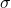-algebra that supports the measure space. But really, what? Thinking about it, it is very difficult to define probability without using the word probable or possible.

Well, probability measures our ignorance about something.

When we make some claim with 90% probability, what we are really saying is that the knowledge we have allows us to make a prediction that is that much accurate. And the main point here is that different people may assign different probabilities to the very same claim! If you have ever seen weather forecasts for the same day disagree, you know what I am talking about. Different data or different models can generate different knowledge, and thus different probability figures.

But we do not have to go that far to find reasonable examples. Let’s consider a very simple one. Imagine you found yourself on a train, and in front of you is sitting a girl with clothes branded Patagonia. What would be the odds that the girl has been to Patagonia? Not more than average, you would guess, because Patagonia is just a brand that makes warm clothes, and can be purchased in several stores all around the world, probably even more than in Patagonia itself! So you would probably say that is surely no more than 50% likely.

But now imagine a kid in the same scenario. If they see a girl with Patagonia clothes, they would immediately think that she had been to Patagonia (with probability 100% this time), because they are lacking a good amount of important information that you instead hold. And so the figure associated with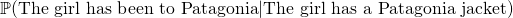is pretty different depending on the observer, or rather on the knowledge (or lack of) they possess. In this sense probability is a measure of our ignorance.

## But WHY is the Lattices Bounded Distance Decoding Problem difficult?

### Introduction to lattices and the Bounded Distance Decoding Problem

A lattice is a discrete subgroup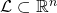, where the word discrete means that each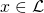has a neighborhood inthat, when intersected with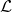results in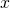itself only. One can think of lattices as being grids, although the coordinates of the points need not be integer. Indeed, all lattices are isomorphic to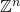, but it may be a grid of points with non-integer coordinates.Another very nice way to define a lattice is: given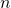independent vectors, the latticegenerated by that base is the set of all linear combinations of them with integer coefficients: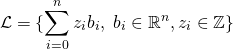Then, we can go on to define the Bounded Distance Decoding problem (BDD), which is used in lattice-based cryptography (more specifically, for example in trapdoor homomorphic encryption) and believed to be hard in general.

Given an arbitrary basis of a lattice, and a point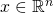not necessarily belonging to, find the point ofthat is closest to. We are also guaranteed thatis very close to one of the lattice points. Notice how we are relying on an arbitrary basis – if we claim to be able to solve the problem, we should be able to do so with any basis.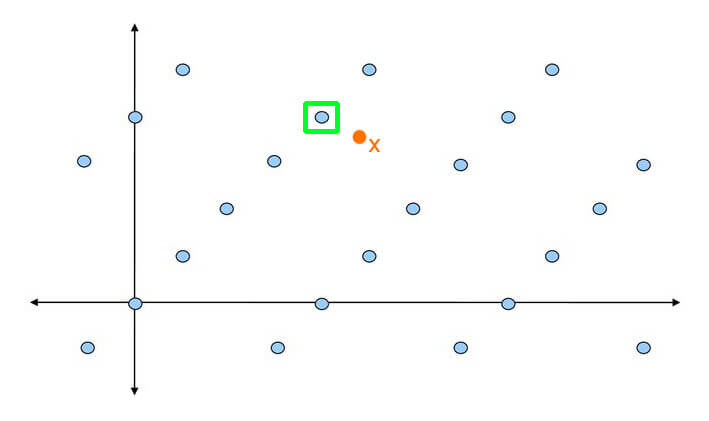Bounded Distance Problem example

Now, as the literature goes, this is a problem that is hard in general, but easy if the basis is nice enough. So, for example for encryption, the idea is that we can encode our secret message as a lattice point, and then add to it some small noise (i.e. a small element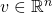). This basically generates an instance of the BDD problem, and then the decoding can only be done by someone who holds the good basis for the lattice, while those having a bad basis are going to have a hard time decrypting the ciphertext.

However, albeit of course there is no proof of this (it is a problem believed to be hard), I wanted to get at least some clue on why it should be easy with a nice basis and hard with a bad one (GGH is an example schema that employs techniques based on this).

So now to our real question. But WHY is the Bounded Distance Decoding problem hard (or easy)?

## Conditional probability: why is it defined like that?

So, you want to calculate the probability of an event knowing that another has happened. There is a formula for that, it is called conditional probability, but why is it the way it is? Let’s first write down the definition of conditional probability: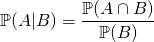We need to wonder: what does the happening of eventtell about the odds of happening of event? How much more likelybecomes ifhappens? Think in terms of howaffects.

Ifandare independent, then knowing something about B will not tell us anything at all about, at least not that we did not know already. In this case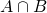is empty and thus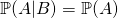. This makes sense! In fact, consider this example: how does me buying a copybook affects the likelihood that your grandma is going to buy a frying pan? It does not: the first event has no influence on the second, thus the conditional probability is just the same as the normal probability of the first event.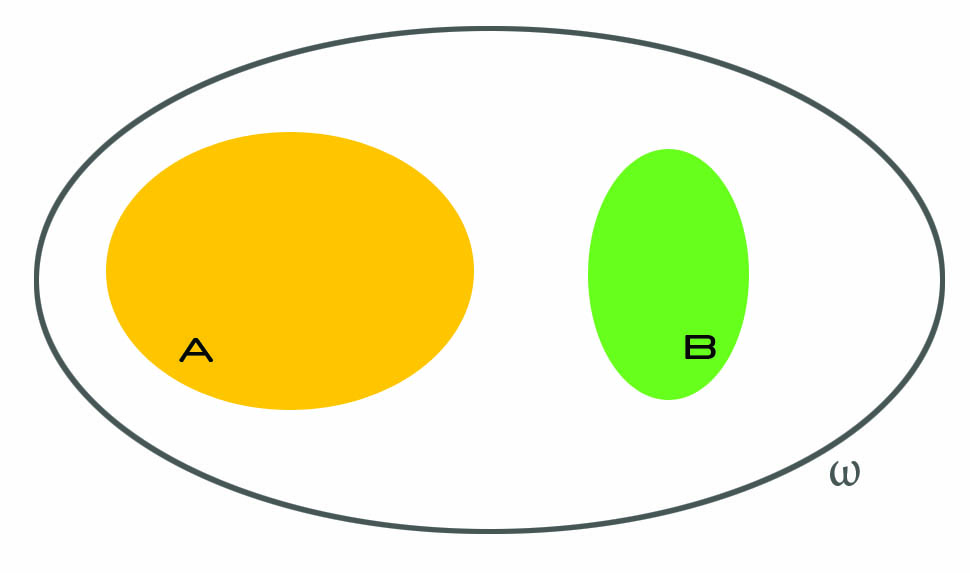Ifandare not independent, several things can happen, and that is where things get interesting. We know that B happened, and we should now think as ifwas our whole universe. The idea is: we already know what are the odds of, right? It is just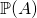. But how do they increase if we know that we do not really have to consider all possible events, but just a subset of them? As an example, think ofversusknowing that all balls are red. This makes a huge difference, right? (As an aside, that is what we mean when we say that probability is a measure of our ignorance.)

So anyway, now we ask: what is the probability of? Well, it would just be, but we must account for the fact that we now live inside, and everything that is outside it is as if it did not existed. Soactually becomes: we only care about the part ofthat is inside, because that is where we live now.

But, there is a caveat. Continue reading “Conditional probability: why is it defined like that?”

## Diagonalizing a matrix NOT having full rank, what does it mean?

This is going to be a quick intuition about what it means to diagonalize a matrix that does not have full rank (i.e. has null determinant).

Every matrix can be seen as a linear map between vector spaces. Stating that a matrix is similar to a diagonal matrix equals to stating that there exists a basis of the source vector space in which the linear transformation can be seen as a simple stretching of the space, as re-scaling the space. In other words, diagonalizing a matrix is the same as finding an orthogonal grid that is transformed in another orthogonal grid. I recommend this article from AMS for good visual representations of the topic.Taken from AMS – We Recommend a Singular Value Decomposition

### Diagonalization on non full rank matrices

That’s all right – when we have a matrix from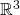in, if it can be diagonalized, we can find a basis in which the transformation is a re-scaling of the space, fine.

But what does it mean to diagonalize a matrix that has null determinant? The associated transformations have the effect of killing at least one dimension: indeed, axmatrix of rank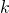has the effect of lowering the output dimension by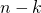. For example, axmatrix of rank 2 will have an image of size 2, instead of 3. This happens because two basis vectors are merged in the same vector in the output, so one dimension is bound to collapse.

Let’s consider the sample matrixwhich has non full rank because has two equal rows. Indeed, one can check that the two vectors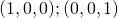go in the same basis vector. This means that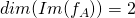instead of 3. In fact, it is common intuition that when the rank is not full, some dimensions are lost in the transformation. Even if it’s axmatrix, the output only has 2 dimensions. It’s like at the end of Inception when the 4D space in which cooper is floating gets shut.

However,is also a symmetric matrix, so from the spectral theorem we know that it can be diagonalized. And now to the vital questions: what do we expect? What meaning does it have? Do we expect a basis of three vectors even if the map destroys one dimension?

Pause and ponder.

## Finding paths of length n in a graph

Suppose you have a non-directed graph, represented through its adjacency matrix. How would you discover how many paths of lengthlink any two nodes?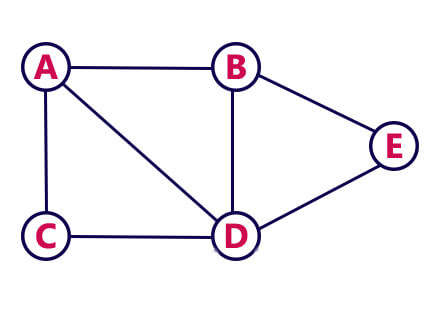For example, in the graph aside there is one path of length 2 that links nodes A and B (A-D-B). How can this be discovered from its adjacency matrix?

It turns out there is a beautiful mathematical way of obtaining this information! Although this is not the way it is used in practice, it is still very nice. In fact, Breadth First Search is used to find paths of any length given a starting node.

PROP.holds the number of paths of lengthfrom nodeto node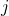.

Let’s see how this proposition works. Consider the adjacency matrix of the graph above: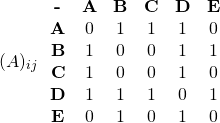With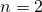we should find paths of length 2. So we first need to square the adjacency matrix:

## On the relationship between L^p spaces and C_c functions for p = infinity

Very quick post on the relationship between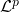,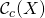and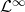. I will assume you already know what I am talking about, I’ll just be sharing some intuition on what those mean, but won’t bother with details. It’s more a reminder for me rather than something that intends to be useful, actually, but there’s almost nothing on the Internet about this!

When we discover that(continuous functions with compact support) is dense in, we also discover that it does not hold if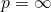and.

What that intuitively means is that if you take away functions infrom, you take away something fundamental for: you are somehow taking away a net that keeps the ceiling up.

The fact that it becomes false for limitless spaces () andmeans that the functions indo not need functions into survive.

This is reasonable: functions inare not required to exist only in a specific (compact) region of space, whereas functions indo. Functions inare simply bounded – their image keeps below some value, but can go however far they want in x direction. Very roughly speaking, they have a limit on their height, but not on their width.

What we find out, however, is that the following chain of inclusions holds:## The meaning of F Value in the Analysis of Variance for Linear regression

This is a sample output for linear regression: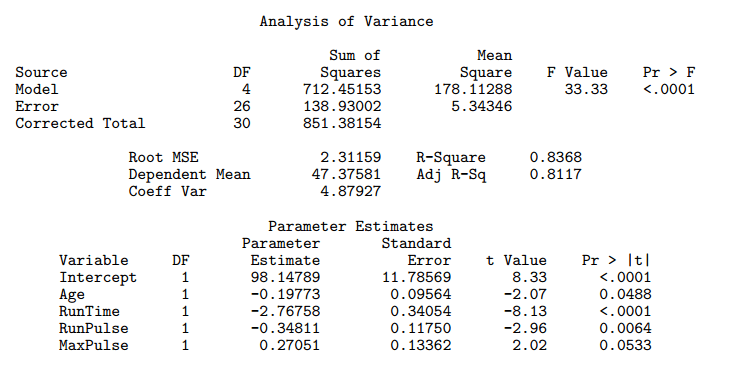The F Value is computed by dividing the value in the Mean Square column for Model with the value in the Mean Square column for Error. In our example, it’s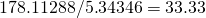.

There are two possible interpretations for the F Value in the Analysis of Variance table for the linear regression.

## On the meaning of hypothesis and p-value in statistical hypothesis testing

Statistical hypothesis testing is really an interesting topic. I’ll just briefly sum up what statistical hypothesis testing is about, and what you do to test an hypothesis, but will assume you are already familiar with it, so that I can quickly cover a couple of A-HAs moments I had.

In statistical hypothesis testing, we

• have some data, whatever it is, which we imagine as being values of some random variable;
• make an hypothesis about the data, such as that the expected value of the random variable is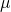;
• find a distribution for any affine transformation of the random variable we are making inference about – this is the test statistic;
• run the test, i.e. numerically say how much probable how observations were in relation to the hypothesis we made.

I had a couple of A-HA moments I’d like to share.

There is a reason why this is called hypothesis testing and not hypothesis choice. There are indeed two hypothesis, the null and the alternative hypothesis. However, their roles are widely different! 90% of what we do, both from a conceptual and a numerical point of view, has to do with the null hypothesis. They really are not symmetric. The question we are asking is “With the data I have, am I certain enough my null hypothesis no longer stands?” not at all “With the data I have, which of the two hypothesis is better?”

## Why hash tables should use a prime-number size

I read in several books and online pages that hash tables should use a prime number for the size. Nobody really justified this statement properly. Here’s my attempt!

I believe that it just has to do with the fact that computers work with in base 2. Just think at how the same thing works for base 10:

• 8 % 10 = 8
• 18 % 10 = 8
• 87865378 % 10 = 8
• 2387762348 % 10 = 8

It doesn’t matter what the number is: as long as it ends with 8, its modulo 10 will be 8. You could pick a huge power of 10 as modulo operator, such as 10^k (with k > 10, let’s say), but

1. you would need a huge table to store the values
2. the hash function is still pretty stupid: it just trims the number retaining only the first k digits starting from the right.

However, if you pick a different number as modulo operator, such as 12, then things are different:

• 8 % 12 = 8
• 18 % 12 = 6
• 87865378 % 12 = 10
• 2387762348 % 12 = 8

We still have a collision, but the pattern becomes more complicated, and the collision is just due to the fact that 12 is still a small number.

Picking a big enough, non-power-of-two number will make sure the hash function really is a function of all the input bits, rather than a subset of them.

## Metaphysics on geometric distribution in probability theory

I realized geometric distribution is not exactly about the time needed to get the first success in a given number of trials. This is a very odd feeling. It is probably a feeling applied mathematicians get sometimes, when they feel they are doing the best they can, and yet the theory is not perfect.

This may be a naive post, I warn you, but I was really stunned when I realized this.

### Geometric distribution is not about the first success

Let’s jump to the point. We know (or at least, I was taught) that geometric distribution is used to calculate the probability that the first success intrials (all independent and of probability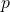) will happen precisely at the-th trial.

Remember that a geometric distribution is a random variable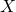such that its distribution is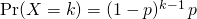How can we relate the above distribution with the fact that it matches the first success? Well, we need to have one success, which explains theat the bottom. Moreover, we want to have just one success, so all other trials must be unsuccessful, which explains the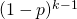.

But hey, where would first ever be written? Continue reading “Metaphysics on geometric distribution in probability theory”